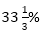SBI Clerk Quantitative Aptitude Quiz: 20th June

Dear Aspirants,

Quantitative Aptitude Quiz For SBI Clerk

Numerical Ability or Quantitative Aptitude Section has given heebie-jeebies to the aspirants when they appear for a banking examination. As the level of every other section is only getting complex and convoluted, there is no doubt that this section, too, makes your blood run cold. The questions asked in this section are calculative and very time-consuming. But once dealt with proper strategy, speed, and accuracy, this section can get you the maximum marks in the examination. Following is the Quantitative Aptitude quiz to help you practice with the best of latest pattern questions.

For Queries related to Adda247 Ultimate for IBPS RRB 2019 Prelims, Click Here or mail us at ultimate@adda247.com

Directions (1-5): Study the table carefully to answer the given questions below.

Q1. What is the average production of wheat in UP throughout all the given years?

1080 quintal
1200 quintal
1000 quintal
980 quintal
1280 quintal

Q2. What is the total production of wheat in 2012 (in quintals)?

4450
4550
4850
5240
4650
Solution:

Total production in
2012 = 1050 + 850 + 1850 + 1100 = 4850 quintal

Q3. What is the difference between total production of wheat by Punjab & Maharashtra over all the given years?

3980 quintals
3500 quintals
2080 quintals
1850 quintals
2250 quintals
Solution:

Required difference = (1250 + 1600 + 1850 + 2400 + 2800) – (850 + 950 + 1100 + 1500 + 2000)
= 9900 – 6400 = 3500 quintal

Q4. What is the ratio between production of wheat by MP in 2011 and 2012 together to the production of wheat in the same years by UP?

37: 33
33: 37
37: 32
32: 37
32: 39

Q5. What is the percentage increase in production of wheat by MP in 2014 than previous year?

Q6. Quantity 1: Average of numbers. Sum of four consecutive even numbers is 76.
Quantity 2: x : x² - 23x + 76 = 0

Quantity I > Quantity II
Quantity I < Quantity II
Quantity I ≥ Quantity II
Quantity I ≤ Quantity II
Quantity I = Quantity II or No relation

Q7. Quantity 1: Length of platform. A train having speed of 72 km/h, crosses a platform and a pole in 24 sec and 8 sec respectively.

Quantity 2: y:Quantity I > Quantity II
Quantity I < Quantity II
Quantity I ≥ Quantity II
Quantity I ≤ Quantity II
Quantity I = Quantity II or No relation

Q8. David can do a piece of work in 16 days. Xavior can do the same work in 24 days. In how many days both will complete the work working together?

8.4 days
8 days
9.6 days
12 days
6 days

Q9. The efficiency of Ruchi ismore than that of Babu. Both complete a work in certain days and get a total wage of Rs. 1680. Find the share of Ruchi in it.

Rs. 720
Rs. 1080
Rs. 860
Rs. 960
Rs. 1260

Q10. 12 men can complete a work in 8 days. 16 women can complete the same work in 12 days. In how many days 10 men and 12 women together can complete the same work?

8 days
6 days
4 days
10 days
2 days

Directions (11- 15): What will come in place of the question mark (?) in the following number series?

Q11. 1015, 508, 255, 129, 66.5, ?, 20.875

34.50
35
35.50
35.75
32.75

Q12. 980, 484, 236, 112, 50, ?, 3.5

25
17
21
29
19

Q13. 32, 36, 52, 88, 152, ?

266
232
242
256
252

Q14. 3, 4, 12, ?, 196

45
40
41
49
56

Q15. 2, 8, 26, ?, 242

78
72
80
84
96
Solution:
Series is × 3 + 2,
× 3 + 2,  × 3 + 2………..

You May also like to Read: